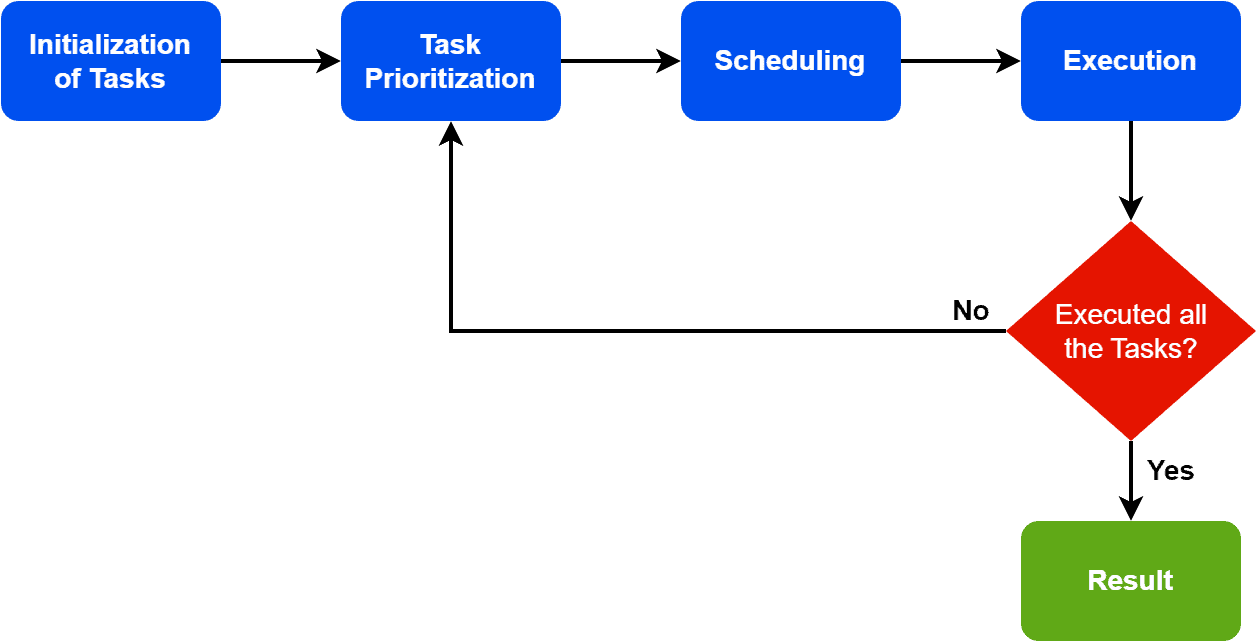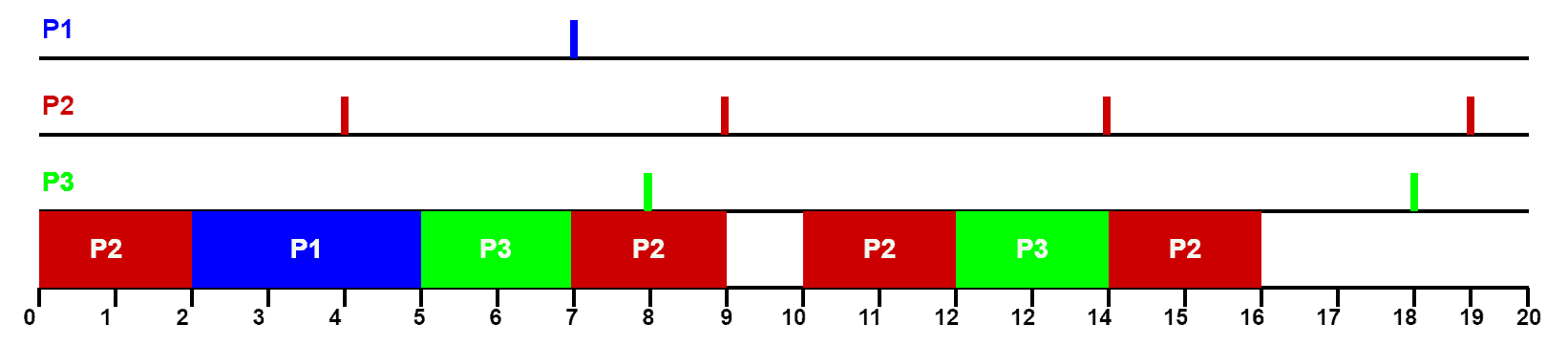## 1. Overview

In this tutorial, we’ll discuss a CPU scheduling algorithm: earliest deadline first. We’ll explain the steps of this algorithm with an example.

## 2. Introduction

Earliest deadline first (EDF) comes under the category of the dynamic scheduling algorithm. We can use it in real-time operating systems for scheduling tasks as well as processes with specific deadlines. It’s a priority-based algorithm where we assign the highest priority to the tasks with the earliest deadline. Additionally, the CPU executes the scheduled tasks based on priority.

The idea behind the EDF scheduling algorithm is to minimize the probability of missing a deadline. Therefore, the algorithm assigns a priority to each task based on its deadline and chooses the task with the earliest deadline for execution. Additionally, if multiple tasks have the same deadline, the CPU executes the task with the highest priority.

EDF is an optimal algorithm for scheduling a set of periodic tasks with known deadlines and execution times as long as the total utilization of the tasks doesn’t exceed unity. Hence, the total execution time of all tasks should be less than the total available time.

It’s a popular scheduling algorithm used in a wide range of real-time applications. We can find its application in distributed, networking, embedded, multimedia, and real-time control systems.

## 3. Working Process

Now let’s discuss how the earliest deadline first scheduling algorithm works. Let’s take a look at the flowchart:The first step is to initialize the available tasks. Additionally, along with initialization, we assign each task a deadline based on completion requirements. The next step is to assign priority to each task. The system sorts the tasks in order of their deadlines, with the task having the earliest deadline assigned the highest priority.

Finally, the CPU executes the selected task until completed or the deadline is reached. If the task is completed before its deadline, the system returns to the task prioritization step to select the next task for execution. If the deadline is reached, the task is considered to be missed.

Now before the algorithm terminates, we need to check two conditions. First, we need to check whether all the tasks are executed. Additionally, we check if all deadlines have been missed. If one of these conditions is met, we need to go back to the task prioritization step and repeat the steps until the algorithm terminates.

EDF is a dynamic scheduling algorithm where the task priority can change over time as deadlines approach. Hence, the algorithm continually updates the priority of tasks based on their deadlines and selects the task with the earliest deadline for execution. Additionally, this helps to ensure that deadlines are met, and tasks are completed in a timely manner.

## 4. Example

Now we know the steps involved in the EDF algorithm. Let’s take an example to demonstrate the algorithm. We’re taking three processes for our example: P1, P2, and P3. Additionally, we assign a capacity, a deadline, and a period for each process:Here, process P1 has a deadline of 7 units of time. Therefore, before the deadline reaches, it must execute 3 units of time over a period of 20 time units. Additionally, we can find similar information for processes P2 and P3 from the table. Now the task is to schedule the processes by considering capacity, deadline, and period:Each line has a marker, which denotes the deadline time for each process. Initially, all the processes are available. We need to pick the process with the earliest deadline compared to others. Here, we can see from the table that process P2 has the earliest deadline. Additionally, as given in the table, the capacity of P2 is 2. Hence, we allocate the first two time units to process P2.

Now there’re two processes available: P1 and P3. Based on the deadline, we assign process P1 which has a capacity of 3 units. Finally, we allocate process P3.

Now at this point, we only need to consider the processes P2 and P3. We already satisfy the allocation requirement for process P1. Between processes P2 and P3, we allocate P2 based on the deadline comparison. Now if we look at the diagram, we need to allocate P2 before the deadline. Hence, we allocate P2 followed by P3. Finally, we allocate P2 again to complete the scheduling.

The EDF is a scheduling algorithm that has several advantages over other scheduling algorithms. Some of the key benefits of this algorithm are guaranteed schedulability, efficient utilization, and dynamic behavior.

EDF is a deterministic scheduling algorithm that provides guaranteed schedulability for real-time systems. Hence, if the system has sufficient processing capacity, it meets the deadlines of all tasks. Therefore, it’s a suitable scheduling algorithm for real-time applications where deadlines are strictly observed.

Another crucial advantage of EDF is that it has the highest utilization among all fixed-priority scheduling algorithms. Therefore, it’s an efficient scheduling algorithm for systems requiring high processing capacity utilization.

Finally, EDF is a dynamic scheduling algorithm. Hence, it adjusts the scheduling of tasks based on the changing demands of the system. It makes EDF suitable for real-time applications where the requirements of the tasks may change over time.